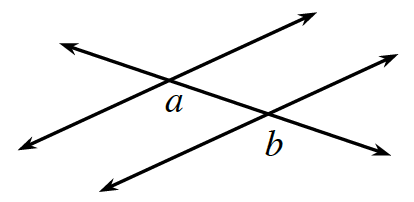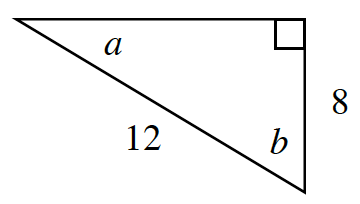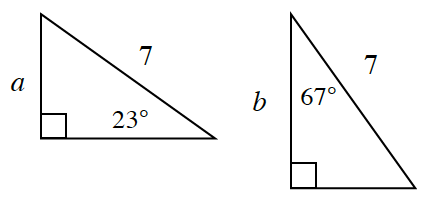### Home > INT2 > Chapter 10 > Lesson 10.1.3 > Problem10-46

10-46.

For each situation below, decide if $a$ is greater, $b$ is greater, if they are the same value, or if not enough information is given.

1. $a$ is the measure of a central angle of an equilateral triangle; $b$ is the measure of an interior angle of a regular pentagon.

The central angle of the triangle is $360°$ divided by $3$, which is $120°$.

1.Is there enough information given?
What do you need to know?

1.Third side is approximately $8.9$ units, so $b$ is opposite the greater side and must be greater than

1. $a = b + 3$

What does this equation tell you?

1.Find the lengths of the unknown sides.